Home  │ Audio Home Page

Updated
March 11, 2010.  See Document History at end for details.

### Introduction

Attempts to get high gain from one class A amplication stage are complicated by the fact that that output DC bias increases with this same gain.  The result is either limited gain or some absurdly large collector/drain power supply.  The obvious solution would be to load the output with an impedance that is sufficiently high in the passband to give the desired gain but low enough at DC to allow reasonable bias voltages.  A sample response is given in figure 1 and the passive circuit model in figure 2.  In the model of figure 2, RP represents the desired passband load, RS sets the DC bias, and L sets the pole (and the zero) to fall below the passband.

 Figure 1:  Bode plot of typical impedance Figure 2:  Passive model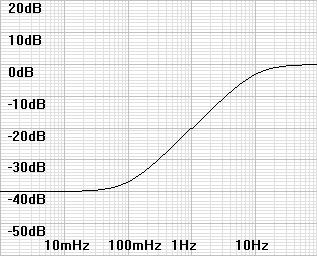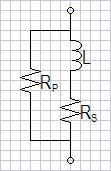It happens that this is the passive equivalent of the simplest of active inductors.  This is fortunate because the passive circuit would require an impossibly large inductor in this application.  Figure 3 shows this active inductor and figure 4 shows its simplified ac model.  If you have another application for an active inductor, remember that the circuit only models an inductor in the sloped region between the zero and the pole.

 Figure 3:  Active Inductor Figure 4:  AC model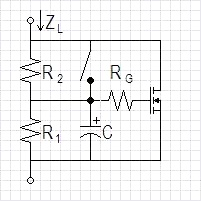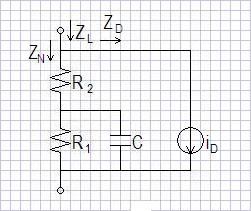iD = gfsvC

### Design Summary

• Choose R2 for gain of overall circuit.
• Calculate/set gfs in preparation for remaining calculations, verifying bounds of equation (22).  (equation (9))
• Choose R1 to set desired DC bias.  (equation (24))
• Choose C to set the pole frequency the desired amount below the passband.  (equation (21))
• Determine if a bypass switch is needed to charge the capacitor on startup.

### Passive Circuit Analysis

As a point of comparison, analyze passive circuit of figure 2.

 (1) Z(s) = RP || ( sL+RS)

 (2) RP(sL+RS) sLRP+RPRS Z(s) ==RP+sL+RS sL+RP+RS

 (3) RPRS RS RS ωZERO ==, fZERO =LRP L 2πL

 (4) RP+RS RP+RS ωPOLE =, fPOLE =L 2πL

### Determination of AC Forward Transconductance

It is necessary to calculate the ac transconductance to continue with the calculations.  Many symbols are used for transconductance such as Gm, gfs, and gm.  I have decided choose and gfs for the ac transconductance, and kn for the transconductance figure of merit in the MOSFET model below.

 (5) MOSFET model1 (6) Simplified model (7) The same solved for v iD = kn(vgs - vT)2 , to simplify calculations let v = vgs - vT, iD = knv2 v = sqrt(iD/kn)

Derive gfs by differentiating the simplifed MOSFET model with respect to v.
 (8) δiD (9) gfs == 2knv ,  substitute v from equation (7): gfs = 2knsqrt(iD/kn), gfs = 2 x sqrt(kniD) δv

If you do not have a specification for kn from which to calculate gfs, you will have to determine it some other way.  Perhaps inspection of a graph.  If you are given gfs, you can rearrange equation (9) to solve for kn.  Furthermore, you may alter the effective gfs for any reason (i.e. gfs parameter stability) by adding a source resistor:

 (10) gfs gfs-effective =gfsRsource+1

### Active Circuit Analysis

It would be easy to presume that the analysis of figure 4 would just be determined from R1, R2, and C.  However this circuit has current feedback from the drain back to the biasing network, and must be analyzed more thoroughly.

 (11) iD = gfs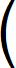R1 || CR1 || C + R2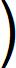vin

,
 (12) iD = gfsvinR1sR1C+1R1+R2 sR1C+1(13) iD = gfsvinR1R1+sR1R2C+R2,
 (14) vin sR1R2C+(R1+R2) ZD ==iD gfsR1

 (15) R1 (16) sR1R2C+(R1+R2) ZN = R2+R1||C  =  R2+, ZN =sR1C+1 sR1C+1

 (17) 1 1 1 (sR1C+1)+gfsR1 YL ==+, YL =ZL ZN ZD sR1R2C+(R1+R2)

 (18) sR1R2C+(R1+R2) ZL =sR1C+(gfsR1+1)

A zero and a pole!

 (19) R1+R2 R1+R2 ωZERO =, fZERO =R1R2C 2πR1R2C

 (20) gfsR1+1 gfsR1+1 ωPOLE =, fPOLE =R1C 2πR1C

### Practical Boundaries to Component Selection

For our application, we want to choose C so that the pole does not affect the passband.  Derive from equation (20):

 (21) gfsR1+1 C =2πfPOLER1

We want ωPOLE >> ωZERO.

 gfsR1+1 R1+R2 gfsR1R2+R2 R1+R2>>,>>, R1C R1R2C R1R2C R1R2C

 gfsR1R2+R2 >> R1+R2 ,  gfsR1R2 >> R1

 (22) gfsR2 >> 1

Any reasonable choice of R2 would likely meet this condition.

### DC Analysis

Our application sets R2 for desired circuit gain.  Now calculate R1 for desired DC bias (vbias).  The gate-source voltage (vgs) driven by the bias current is multiplied by the R1-R2 resistor divider.  The first equation is the product of the resistor divider factor and the MOSFET equation (5) solved for vgs.  Note that iD here is the DC drain current.

 (23) vbias =R1+R2R1vT+sqrt(iD/kn)R1vbias R1+R2 =,  R2 = R1 vT+sqrt(iD/kn)vbias-1 vT+sqrt(iD/kn)(24)

R2

R1 =vbias-1 vT+sqrt(iD/kn)If you used a source resistor to modify gfs add the source resistor voltage to vT in these equations:

 (25) vT-effective = vT + iDRsource

If vT >> sqrt(iD/kn)  (a fairly common assumption), an approximate DC analysis is acceptable instead of equation (24).
(26)

R2

R1 =vbias vT -1### Example to come...

1Donald L. Schilling, Charles Belove, Electronic Circuits:  Discrete and Integrated (New York, 1979), p. 143 equation (3.2-2b)

Document History
October 21, 2009  Created.
October 23, 2009  corrected equation (21).
March 3, 2010  Added equation (26) for approximate DC analysis.
March 11, 2010  Changed designation for MOSFET constant to proper kn.